# Lens–9th Standard Science Question Bank

Lens

1. A transparent material which is thick in middle and thinner at the edges is called ---

a) Concave lens b) Convex lens

c) Plano-concave lens d) convex-concave lens

2. Where should be an object be placed in front of a convex lens to get a real image of the size of the object?

a) at infinity

b) at the principal focus

c) at twice the focal length

d) between the optic center & principal focus

3. The image of an object formed by a concave lens is

a) Virtual, inverted & diminished b) Virtual, upright & diminished

c) Virtual, inverted & enlarged d) Virtual, upright & enlarged

4. An object is placed at a distance of 5cm from a convex lens of focal length 10 cm. The image formed is -----

a) real & inverted b) real & enlarged

c) virtual & diminished d) virtual & enlarged

Fill in the blanks with suitable words

1. A ray of light passes undeviated after refraction from a thin lens when it is incident at ----- (principal axis)

2. Virtual, enlarged & erect image is formed on the same side of an object in the convex lens, then the position of the object is --------- (between F and O)

3. The distance between principal focus & the optic centre of a lens is called its ------- (focal length)

Match the followings:

1.The centre point of a lens - Optic centre

2. Diverging lens - Concave lens

3. The distance between principal focus & the optic centre of a lens  - focal length

4. the images caught on the screen - real image

1. What is a lens?

Lens is a piece of transparent material bounded by one or more curved surfaces.

2. Name types of lenses.

Convex lens, concave lens, Plano convex lens & Plano concave lens.

3. Write differences between convex lens & concave lens.

a) Convex lens is thicker at middle than at edges but concave lens is thinner at middle than at edges.

b) Convex lens converges light rays but concave lens diverges light rays.

c) Convex lens is called as converging lens but concave lens is called as diverging lens.

4. Explain with a ray diagram, why the convex lens is also called a converging lens.

Convex lens converges rays of light falling on it.  Hence it is called converging lens.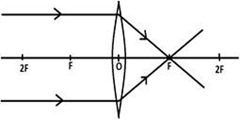5. Define aperature of lens.

The surface of lens through which refraction takes place is called aperature of lens.

6. What is optic centre of lens?

The centre point of lens is called optic centre of lens. Optic centre of lens is denoted by ‘O’

7. Define principal axis of a lens.

The line passing through optic centre of the lens & perpendicular to aperture is called principal axis.

8. What is principal focus of lens?

The point on principal axis at which rays of light converges or appears to be diverges is called principal focus of lens. It is denoted by the symbol ‘F’.

9. What is focal length?

The distance between optic centre & principal focus is called focal length/ it is denoted by the symbol ‘f ’.

10. What is the speed of light in vacuum?

Speed of light in vacuum is 3 lakh kilometer per second.

11. What is real image?

The image that can be obtained on screen is called real image.

12. What is virtual image?

The image that cannot be obtained on screen is called virtual image.

13. Write the lens formula .

1/f = 1/u + 1/v

Here v = image distance

u = object distance

f = focal length.

14. Explain with a ray diagram, why the concave lens is also called a diverging lens.

Concave lens diverges rays of light falling on it.  Hence it is called diverging lens.

15. Define principal focus of a convex lens & that of a concave lens.

The point on principal axis at which rays of light converges (in case of convex lens) or appears to be diverges (in case of concave lens) is called principal focus of lens. Principal focus is denoted by the symbol ‘F’.

16. Draw a diagram to show how a converging lens is able to form the image of the sun.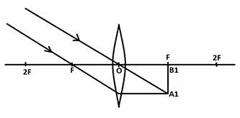17. An object is placed beyond twice the focal length of a convex lens. Find the position nature and size of the image with the help of a ray diagram

When an object is placed beyond twice the focal length of a convex lens, nature of the image obtained is real , diminished and inverted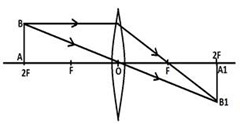18. List the position of object, position of image, nature of image and practical applications in different situations.

 Ray diagram position of object position of image nature & size of image practical applications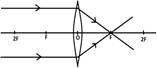at infinity at F real, inverted &highly diminished telescope objective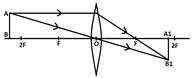beyond 2F between F & 2F real , diminished inverted camera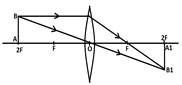at 2F at 2F real,same size of the object inverted telescope objective erecting lens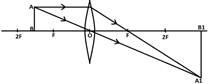between F & 2F beyond 2F real, enlarged, inverted projector,objective of a microscope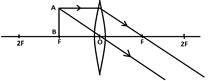at F at infinity real,infinately large, inverted spot lights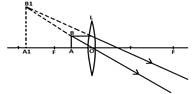between F & O on the same side of the object virtual, enlarged,erect magnifying lens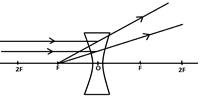at infinity at F virtual,erect,highly diminished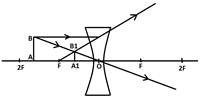between infinity & O between F & O virtual,erect, diminished